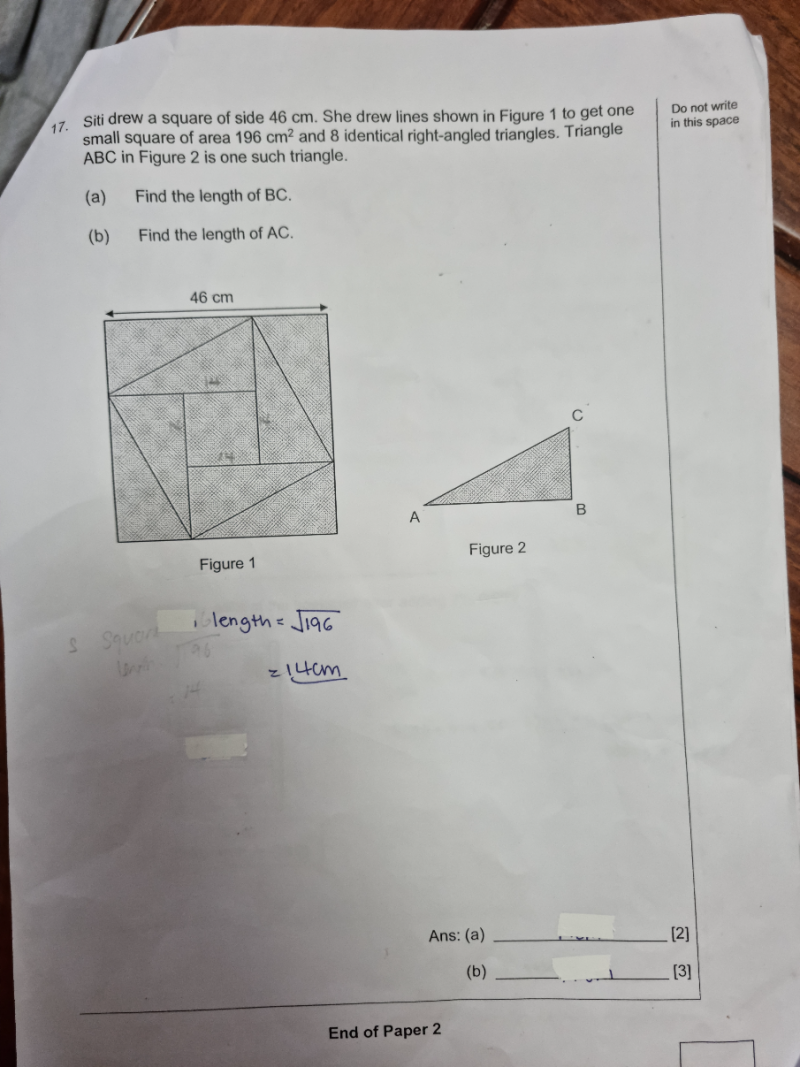# QuestionThank you

(a) 2BC + 14 = 46  => 2BC = 32  => BC = 16cm

(b) AB = 14 + 16 = 30

Area of right angle triangle = 1/2 (30)(16)  = 240

Area of 4 right angle triangles = 240 x 4 = 960

Area of the square inside = (46 x 46) – 960 = 2116 – 960 = 1156

Hence AC = sqrt(1156) = 34cm